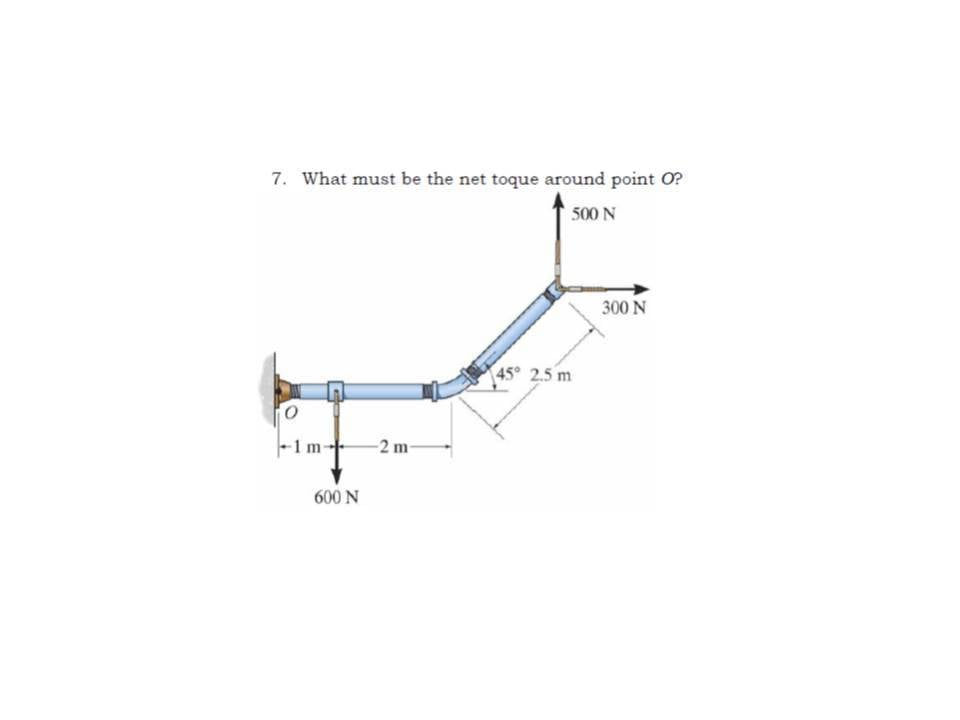# Net Torque on an Angled Arm

draupe

## Homework StatementMy teacher gave us a solution of + 1250 Nm Where CCW = positive torque
I know that the torque of the 600N + 300N forces + 1250Nm = the torque of the 500N force.
I can't figure out what angles work with the forces at the end of the pipe.

Σ T = 1250 Nm
T =Fr sinΘ

## The Attempt at a Solution

ΣT = (500N x5.5m) - (600N x 1m) - (300N x 5.5m x sin 45°)

ΣT = (2750Nm) - (600Nm) - (1166.7 Nm)

ΣT = +983.3 Nm This is the answer I get when I attempted the problem.

I've also tried setting those forces with the hypotenuse as the r for the 500 and 300 N forces.
The triangle would be 4.58m base, 1.58m height. Pythagoras would say this triangle's last side( hypotenuse) would be 4.84m.

Also I tried sin of 135° turns out it is equivalent to sin 45°

ΣT = (500N x 4.84m) - (600N x 1m) - (300N x 4.84 x sin 45°)

ΣT = 2420Nm - 600Nm - 1026.7 Nm

ΣT = 793.3 Nm

#### Attachments

•